String Annotations for Plots

Did you know that you can customize the direction of text in MATLAB plots? There are times when such annotations work better than if they were purely horizontal or vertical.

Sunspot Data

Let's look at some sunspot data. First I'll load it into MATLAB.
Separate the year from the actual number of sunspots.
year = s(:,1);
spots = s(:,2);
Show the data in a bar plot.
bar(year,spots)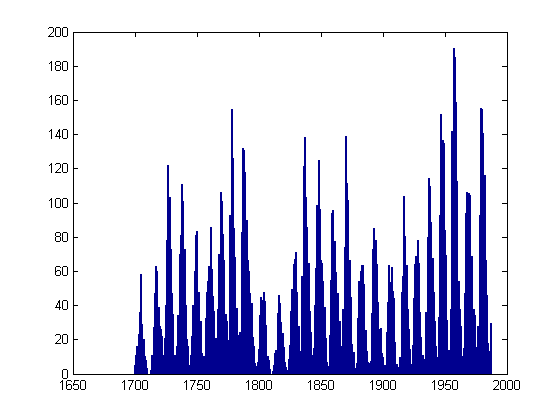Annotate the Year with the Maximum Number of Sunspots

[smax, indmax] = max(spots);
The historical record shows the year
yearmax = year(indmax)
yearmax =
1957
with the maximum number of sunspots, 190.
hold on
plot(yearmax, smax, 'm*')
hold off
ht = text(yearmax, smax,['  ',int2str(round(smax)), ' sunspots  ']);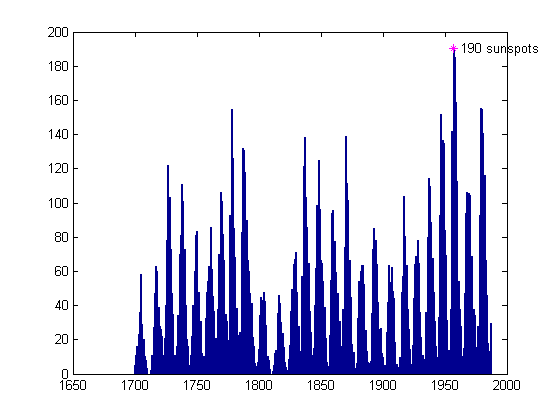Use Handle Graphics to Alter the Annotation Orientation

Let's try labeling the maximum so the label is right-justified now.
set(ht,'HorizontalAlignment','right')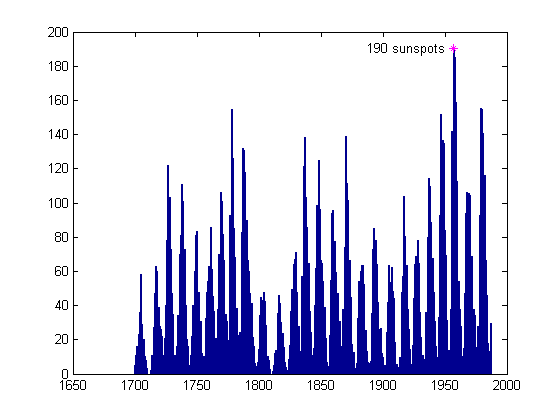Now change the label so it is at an angle, something I might want if I were planning to label several more points on the graph.
set(ht,'Rotation',45)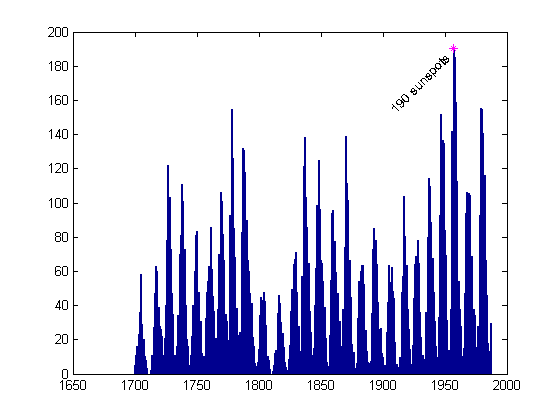Note Other Maxima

The sunspot cycle has a periodicity of about 11 years. Let's see which other years have a high number of sunspots.
[maxssa, indssa] = sort(spots,'descend');
year(indssa(1:10))
ans =
1957
1958
1959
1979
1980
1778
1947
1956
1981
1870
We see some years near the maximum year, 1957, then another cluster near 1979, and the next at 1778. Let me grab the first five "distinct" clusters.
idxdistinct = [1 4 6 7 8];
year(indssa(idxdistinct))
ans =
1957
1979
1778
1947
1956

Label Extra Peaks

Let's label these few other peaks in a similar manner to the first one.
nearMaxYears = year(indssa(idxdistinct(2:end)))
ssaMax = spots(indssa(idxdistinct(2:end)))
hold on
plot(nearMaxYears, ssaMax, 'mo')
hold off
hn = text(nearMaxYears, ssaMax,['  high sunspot activity  '],...
'HorizontalAlignment','Right','Rotation',25);
set(ht,'Rotation',0)
nearMaxYears =
1979
1778
1947
1956
ssaMax =
155.4000
154.4000
151.6000
141.7000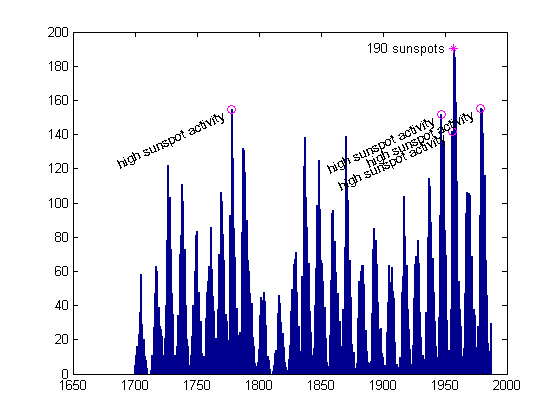Write Around a Circle

Just for fun now, let's write a phrase around a circle of radius 1.
clf, box on
phrase = 'ring around the collar ';
num = length(phrase)
angles = 0:(360/num):359;
x = cos(angles*pi/180);
y = sin(angles*pi/180);
for ind = 1:length(phrase)
text(x(ind),y(ind),phrase(ind),'Rotation',angles(ind),...
'HorizontalAlignment','center')
end
axis equal, axis([-1.1 1.1 -1.1 1.1])
num =
23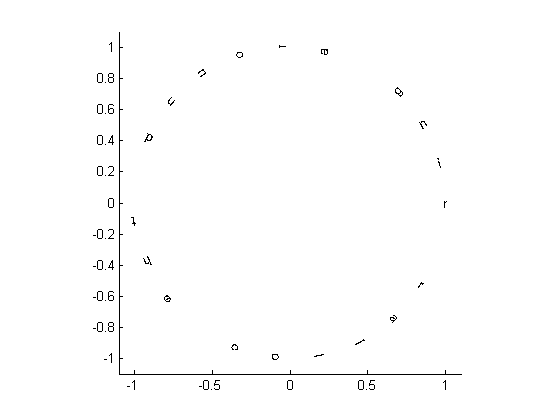References

There is a whole lot more you can do with text annotation in MATLAB. Here are some references for the language aspects of working with text. Though I don't want to turn this blog into one chiefly about graphics, I think I can cover some aspects here and still talk about it in terms of the language. Any suggestions? Please post them here.

Published with MATLAB® 7.4
|